## Colouring Above or Below an Average Line

by Harriet Owen

Adding context to graphs is good practice because it can help the user understand more about what the data points mean faster. One method is to add colour to show whether a data point sits above or below a specific reference value and this can be achieved with two slightly different methods, which I will outline below.

In the following example I will be using the Sample - Superstore dataset. I will demonstrate how the total Sales for each Region by Category sit above or below the average Sales within that Region.

First, I built my view to show the total Sales for each Region by Category. I did this by dragging Region and Category onto the rows shelf and Sales onto the columns shelf.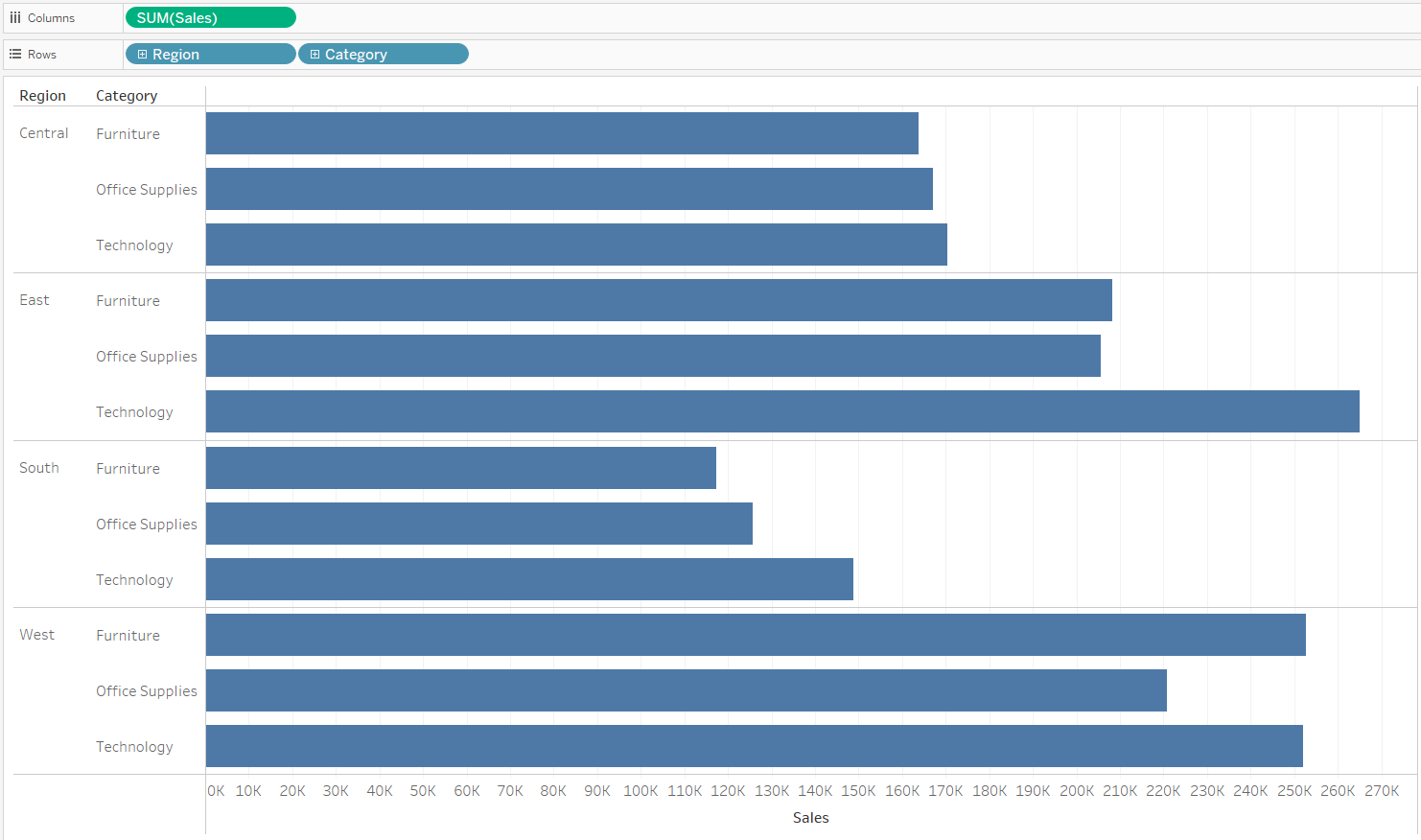Initial sum of sales for each region by category view.

Next I added an average line (from the analytics pane) onto my view, ensuring this was added at the pane level. I then edited this so that my label was 'value' and not the default 'computation'.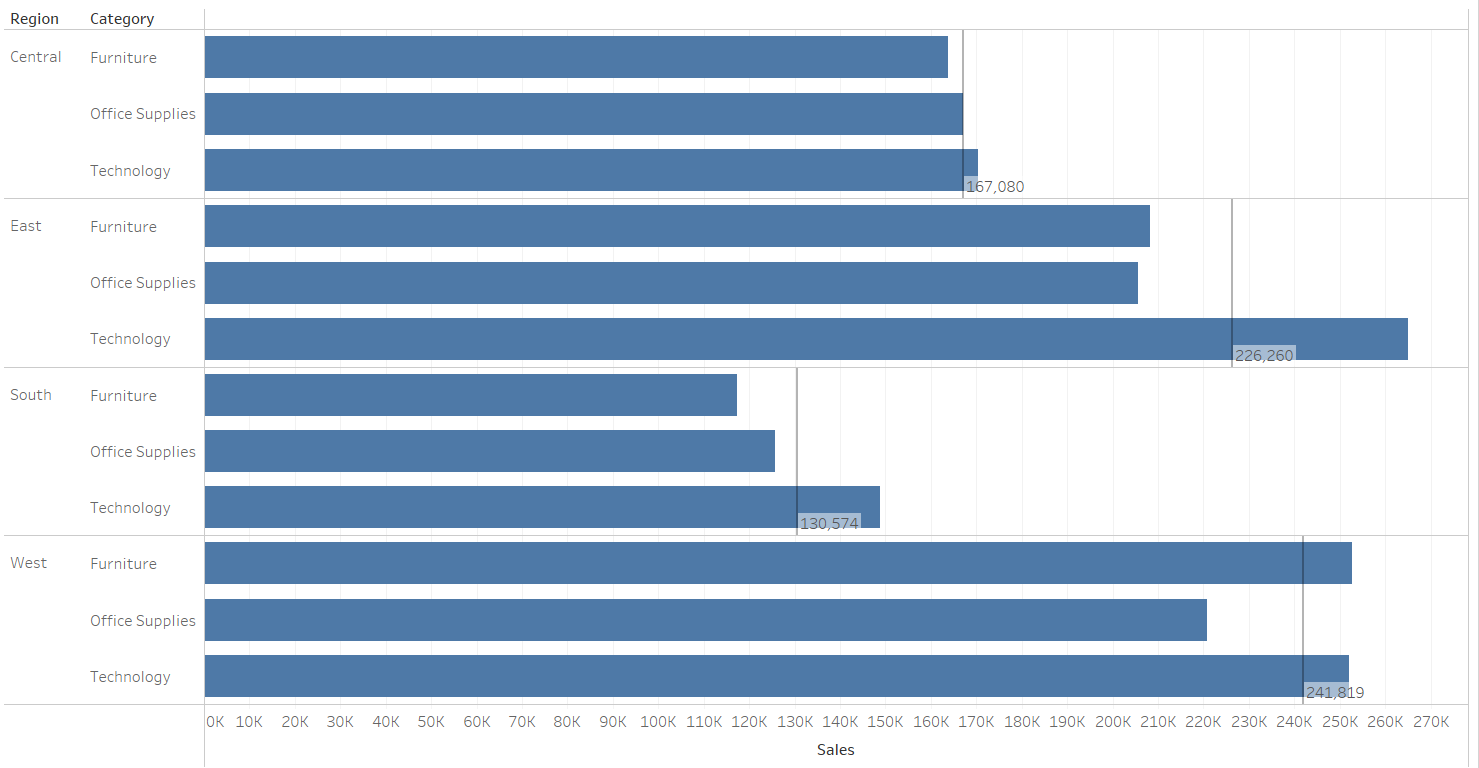Average line per pane added.

Now that the view is set up, the last step was to add colour to show which Categories within each Region have a total Sales greater than the average Sales within that Region. Two methods exist to achieving this:

1. Use a table calculation

This required the following steps:
a) I first calculated the average Sales per Region using a table calculation known as Window AVG, as shown below: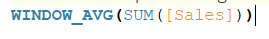Table Calculation - Average Region Sales

b) Then I used the above calculation to specify if Sales per Region and Category were greater than the average Region Sales. If they were, the result returned was true and if not, a false was returned (as shown below).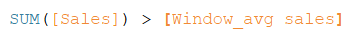Above or Below Average Region Sales

c) Finally, I used this latest calculation and placed it on colour within the marks card. This will likely not colour your bars correctly and this is due to the default settings of a table calculation.

Table calculations can be configured differently depending on what is present within the view. In this scenario, when I add the above calculated field to colour on the marks card I can right click and edit the table calculation. This presents the following view:

Here you can specify how you would like the calculation to be computed. In this example, I wanted to calculate the average Sales for each Region by Category. As a general rule of thumb your 'each' field (Region) is what you want to uncheck and the 'by' field (Category) is what you want to check. I set up my table calculation to correctly calculate the average sales per region by selecting 'Specific Dimensions' and only checking Category.

The end result after the above adjustments looked like this: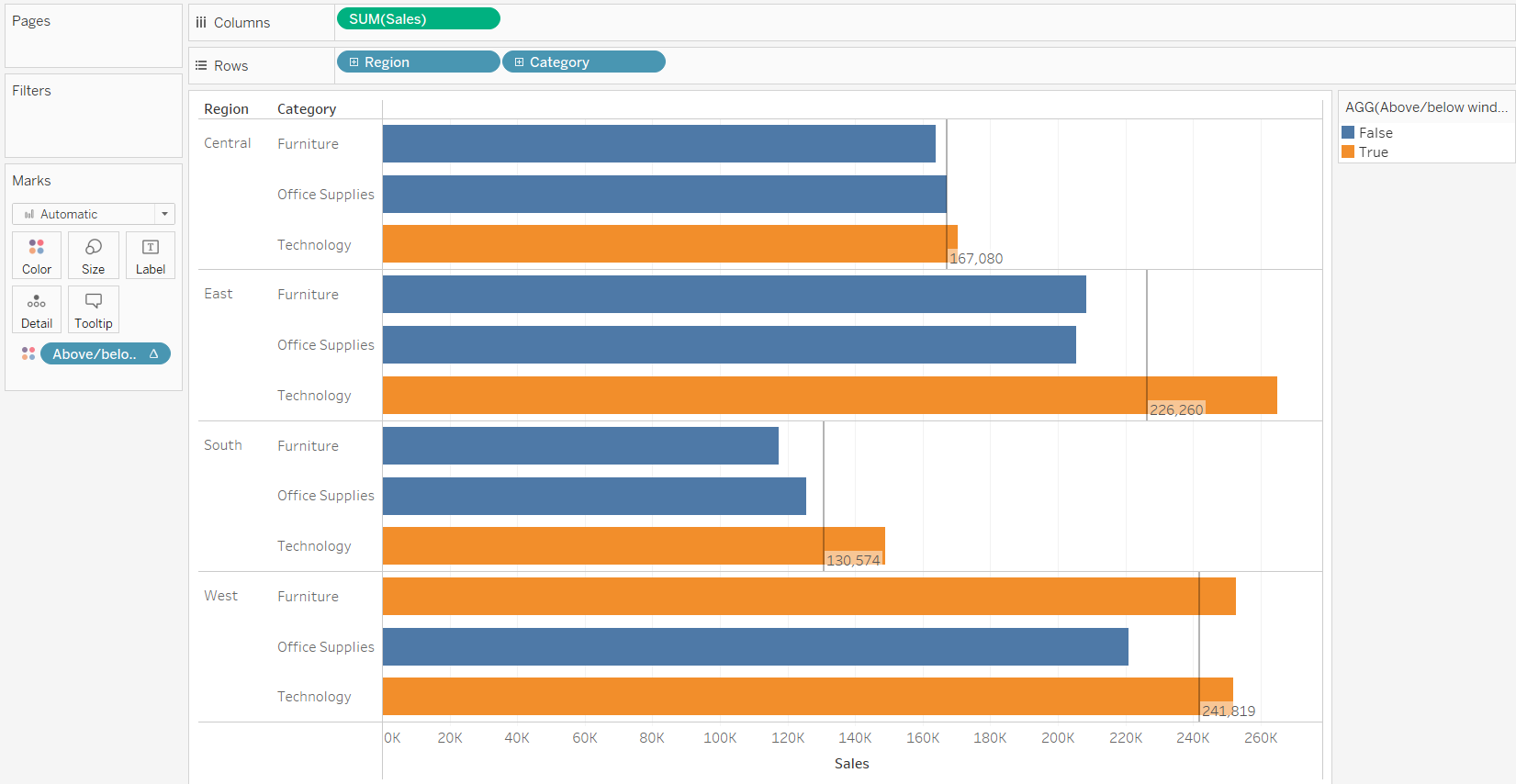Final result using Window AVG

2. Use a nested LOD calculation

This required the following steps:
a) Similar to the first step in the table calculation approach, I first calculated the average Sales per Region. However, the difference in this approach is I used a nested Level of Detail (LOD) calculation. This first calculated the sum of Sales for Category within each Region and then took the average of those sum values, as shown below: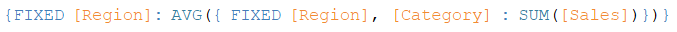Nested LOD- Average Region Sales

b) Again, I then used the above calculation to specify if Sales per Region and Category were greater than the average Region Sales. If they were, the result returned was true and if not, a false was returned (as shown below).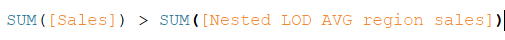Above or Below Average Region Sales

c) Finally, I placed this last calculation onto colour within the marks card and achieved the same output as the Window AVG option.

Which to use?
As shown, both return the same overall result. However, it is good practice to future proof your work for any updates to your view. An LOD fixes the view to the fields you specified and so therefore, any fields added to the view will not update the average calculated. Overall, this makes the view less dynamic. In comparison, a Window AVG will take into consideration whatever is in view, added or removed. Therefore, when possible I would recommend choosing the Window AVG option.Harriet Owen

Wed 02 Jun 2021

Thu 13 May 2021

Wed 05 May 2021

Fri 07 May 2021

© 2021 The Information Lab Ltd. All rights reserved.